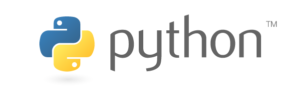# All You Need to Know about Linear Search in Python

Last updated on Apr 24,2020 431 ViewsPYTHON is one of the trending programming application now. Unlike C language it does not require many of the following commands and is made as much as user-friendly as possible. In this article, we will understand Linear Search in python in the following sequence:

## Types of Searches

Basically, There are two types of searches.

In this blog, only linear search is discussed. Linear search Being one of the most popular and basic programs that the beginners learn. The below-given program executes the linear search. Linear search is searching a given number in an array of numbers that the user gives and printing the given position of the number out of all the inputs given.

## What is a Linear Search?

A linear search, also known as a sequential search, is a method of finding an element within a list.It checks each element of the list sequentially until a match is found or the whole list has been searched.

## Code for Linear Search

```lst = []

num = int(input("Enter size of list: t"))
for n in range(num):

numbers = int(input("Enter the array of numbers: t"))
lst.append(numbers)
x = int(input("nEnter number to search: t"))
found = False
for i in range(len(lst)):
if lst[i] == x:
found = True
print("n%d found at position %d" % (x, i+1))
break
print("n%d is not in list" % x)
input()
```

## Function Explained

• Enter the size of the array.

• Press enter key after entering each input.

• Enter the array of numbers.

• Enter the number to be searched.

• The given position starting from numerical 1  will be displayed.

• If the number is not present in the list then the“number not found in the list” statement will be executed.

• The result of the given program can be seen at the end of the program at the execution.

With this, we come to an end of this article on Linear Search in Python. I hope you’ve got a clear idea of how to use Linear Search.

If you found this article on “Linear Search In Python” relevant, check out the Edureka Python Certification Training, a trusted online learning company with a network of more than 250,000 satisfied learners spread across the globe.

We are here to help you with every step on your journey and come up with a curriculum that is designed for students and professionals who want to be a Python developer. The course is designed to give you a head start into Python programming and train you for both core and advanced Python concepts along with various Python frameworks like Django.

If you come across any questions, feel free to ask all your questions in the comments section of “Linear Search In Python” and our team will be glad to answer.REGISTER FOR FREE WEBINARThank you for registering Join Edureka Meetup community for 100+ Free Webinars each month